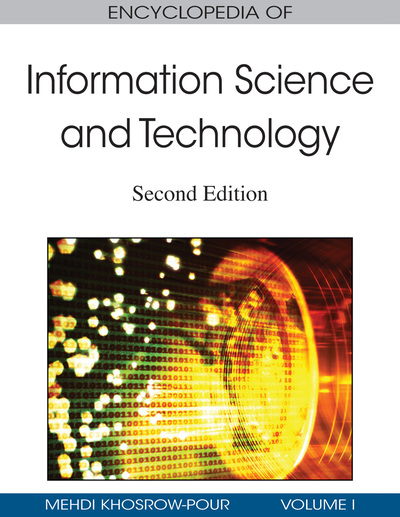# Predictive Data Mining: A Survey of Regression Methods

Sotiris Kotsiantis (University of Patras, Greece and University of Peloponnese, Greece) and Panayotis Pintelas (University of Patras, Greece and University of Peloponnese, Greece)
DOI: 10.4018/978-1-60566-026-4.ch495
Available
\$29.50
No Current Special Offers

## Abstract

Data mining is the extraction of implicit, previously unknown, and potentially useful information from data. The idea is to build computer programs that sift through databases automatically, seeking regularities or patterns. Strong patterns, if found, will likely generalize to make accurate predictions on future data. Machine learning (ML) provides the technical basis of data mining. It is used to extract information from the raw data in databases—information that is expressed in a comprehensible form and can be used for a variety of purposes. Every instance in any data set used by ML algorithms is represented using the same set of features. The features may be continuous, categorical, or binary. If instances are given with known labels (the corresponding correct outputs), then the learning is called supervised in contrast to unsupervised learning, where instances are unlabeled (Kotsiantis & Pintelas, 2004). This work is concerned with regression problems in which the output of instances admits real values instead of discrete values in classification problems.
Chapter Preview
Top

## Background

A brief review of what ML includes can be found in Dutton and Conroy (1996). A historical survey of logic and instance-based learning is also presented in De Mantaras and Armengol (1998). The first step of predictive data mining is collecting the data set. If a requisite expert is available, then he or she can suggest which fields (attributes, features) are the most informative. If not, then the simplest method is that of “brute force,” which means measuring everything available in the hope that the right (informative, relevant) features can be isolated. However, a data set collected by the brute-force method is not directly suitable for induction. It contains, in most cases, noise and missing feature values, and therefore requires significant preprocessing (Zhang, Zhang, & Yang, 2002). Hodge and Austin (2004) have recently introduced a survey of contemporary techniques for outlier (noise) detection. Depending on the circumstances, researchers have a number of methods to choose from to handle missing data (Batista & Monard, 2003). Feature subset selection is the process of identifying and removing as many irrelevant and redundant features as possible (Yu & Liu, 2004). The fact that many features depend on one another often unduly influences the accuracy of supervised ML models. This problem can be addressed by constructing new features from the basic feature set (Markovitch & Rosenstein, 2002).

The problem of regression consists of obtaining a functional model that relates the value of a target continuous-variable y with the values of variables x1, x2...xn (the predictors). This model is obtained using samples of the unknown regression function. These samples describe different mappings between the predictor and the target variables. The traditional approach for prediction of a continuous target is the classical linear least-squares regression (Fox, 1997). The model constructed for regression in this traditional approach is a linear equation. By estimating the parameters of this equation with a computationally simple process on the training set, a model is created. However, the linearity assumption between input features and predicted value introduces a large bias error for most domains. That is why most studies are directed to nonlinear and nonparametric techniques for the regression problem.

## Key Terms in this Chapter

Data Cleansing: This is the process of ensuring that all values in a data set are consistent and correctly recorded.

Nearest Neighbor: It is a technique that predicts the value of each record in a data set based on a combination of the values of the k record(s) most similar to it.

Regression Analysis: It is a technique that examines the relation of a dependent variable to specified independent variables.

Artificial Neural Networks: They are nonlinear predictive models that learn through training and resemble biological neural networks in structure.

Rule Induction: It is the extraction of useful if-then rules from data based on statistical significance.

Predictive Model: A predictive model is a structure and process for predicting the values of specified variables in a data set.

## Complete Chapter List

Search this Book:
Reset